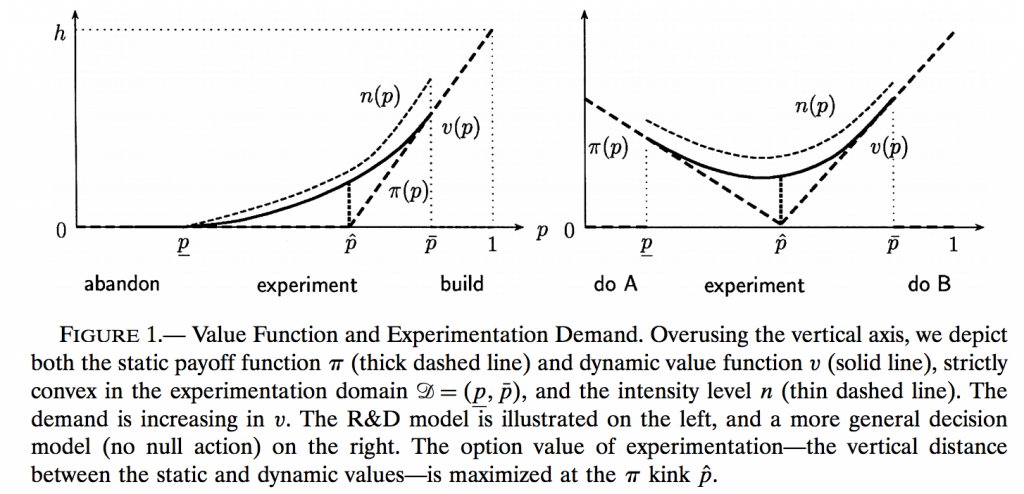# The Optimal Level of Experimentation

“The Optimal Level of Experimentation, Moscarini and Smith (Econometrica, 2001)

PRESENTATION SLIDES (albeit 1990s vintage)

MY CLASS NOTES TO TEACH THE PAPER

Assuming two states and two actions. Imagine that an decision maker like a firm can experiment at a variable rate before choosing an irreversible action. If the decision maker were perfectly patient, and information had a constant marginal cost “per signal”, then this is a long-solved problem by Wald: Experiment until you are sure enough of one state or the other. But towards an element of realism, assume the firm is impatient. In this case, the firm would wish to experiment at an unboundedly high intensity until we hit this “sure enough” stopping set. But this is not realistic. What it misses is that independent Bayesian signals are generally not produceable at a high rate. We assume an increasing, strictly convex cost before choosing an irreversible action. We do so by modeling information purchase as a control of variance of a diffusion with uncertain mean. The level of experimentation is the precision of this signals.

In this environment, the underlying static payoffs are the V-shaped decision as a function of the state variable, which is the belief in the high state. The optimal value is smoothly pasted onto this frontier (hat tip: Samuelson, 1965):We prove: (a) the optimal experimentation level rises in the Bellman value; and deduce testable implications, like (b) experimentation costs drift up; and (c) a more impatient decision maker may experiment more, given lump-sum final payoffs. We show that (a) and (b) are robust to finitely many states and actions, and we also extend an R&D interpretation of the model, where experimentation is monotonic not only in the value, but also in beliefs.

An interesting fact about this paper: Our Proposition 5 asserts that as the interest rate r rises, the optimal experimentation level n rises strictly near the extremes. In other words, the standard intuition that R&D expenditures should fall as the discount rate rises is false. For it ignores the fact that an impatient decision maker might wish to quickly finish his project. This one result of ours was later rediscovered by Rja Chetty in Interest Rates, Irreversibility, and Backward-Bending Investment Review of Economic Studies 74(1): 67-91, 2007. (I mention this, as we are not cited there.) He found that “At low rates, increasing the interest rate raises investment by increasing the cost of delay.” Our framework affords a wealth of predictions aside from this one. For instance, on the time series structure, if the producer surplus is concave, then  experimentation level drifts upward.